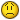Using value in two cells as the range for a MAX function

M

Mark

Hi there,

So I have this type of data, but much more of it than I copied:

Time Position Torque
0 121.3 2.71
0.01 121.3 3.25
0.02 121.1 3.93
0.03 120.9 4.34
0.04 120.8 4.88
0.05 120.6 5.42
0.06 120.3 6.1

I am trying to search a specific range of the the data for a maximum in the
torque column, for example "what is the maximum torque between .5 and 1.5
seconds". I have used the Match function to give me the row numbers that I
want to search (say, .5 seconds is row 50 and 1.5 seconds is row 150) I would
type in "=MAX(C50:C150) to find the maximum torque between those times. Is
there a way to have the MAX function refer to the rows that I have had excel
find? Here is what I have so far:

A B
1 Min Max
2 0 1.228
3 6 128
4 C6 C128

Here I am trying to have excel find the maximum torque between 0 seconds and
1.228 seconds. The match function tells me that 0 seconds is row 6 and 1.228
seconds is row 128, thus I want to find the maximum in C6 to C128. How can i
do this without manually writing the search range each time? Perhaps
something like "=MAX((=A4)=B4))"? I tried that with no luck.

I hope you can understand the question! It is difficult to explain. Thanks!

Mark

G

Gary''s Student

=MAX(INDIRECT(A4 & ":" & B4))

so if A4 contains C8
and if B4 contains C20
and C1 thru 30 contains:

1
2
3
4
5
6
7
8
9
10
11
12
13
14
15
16
17
123
19
20
21
22
23
24
25
26
27
28
29
999

then the formula returns 123

J

Jacob Skaria

Dear Mark

For maximum torque between .5 and 1.5

D1 = 0.5
E1 = 1.5

Please note that this is an array formula. Within the cell in edit mode (F2)
paste this formula and press Ctrl+Shift+Enter to apply this formula. If
successful in 'Formula Bar' you can notice the curly braces at both ends like
"{=<formula>}"

=MAX(IF(INDIRECT("A"& LOOKUP(D1,A2:A100,ROW(A2:A100))
&":A100")<=E1,INDIRECT("C" & LOOKUP(D1,A2:A100,ROW(A2:A100)) & ":C100")))

Try and feedback...

If this post helps click Yes

T

T. Valko

Array entered** :

E2 = lower boundary = 0.5
F2 = upper boundary = 1.5

=MAX(IF((A2:A10>=E2)*(A2:A10<=F2),C2:C10))

** array formulas need to be entered using the key combination of
CTRL,SHIFT,ENTER (not just ENTER). Hold down both the CTRL key and the SHIFT
key then hit ENTER.

The sample data that was posted shows the torque as an increasing value. If
that pattern holds true throughout the data range then all you need to do is
a lookup on the upper boundary.

=VLOOKUP(F2,A2:C10,3,0)

Maybe even:

=SUMIF(A2:A10,F2,C2:C10)